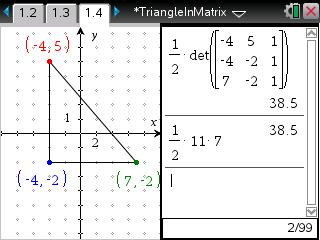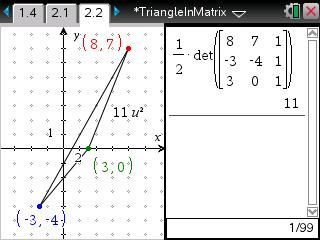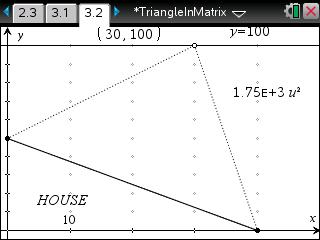# Activities

••• ##### Subject Area

• Math: Algebra II: Matrices

• ##### Author9-12

15 Minutes

• ##### Device
•TI-Nspire™ CX/CX II
•TI-Nspire™ CX CAS/CX II CAS
• TI-Nspire™
• TI-Nspire™ CAS
• ##### Software

TI-Nspire™
TI-Nspire™ CAS

3.2

## Triangle in the Matrix

#### Activity Overview

In this activity, students will represent a triangle with a matrix and use the determinant to find the area of three given triangles.

#### Key Steps

•In this activity, students will find the area of a right triangle using a matrix and verify the formula using A = 0.5bh.

•Then students will practice using the matrix formula and confirm their answer by finding the area of the triangle on the Graphs application.

•In the extension, students are asked to find the vertex of a triangle given the other two vertices and the area. They can solve this problem algebraically or use a Graphs page.